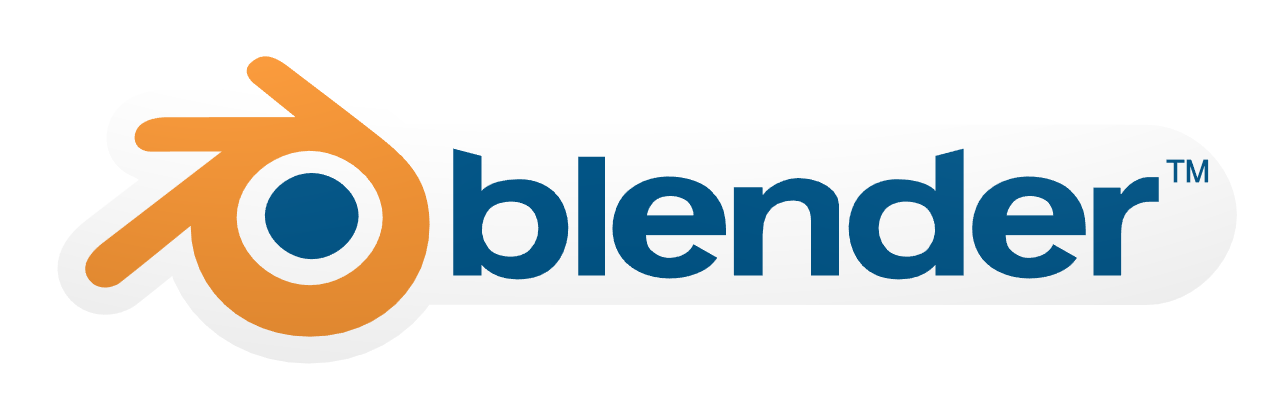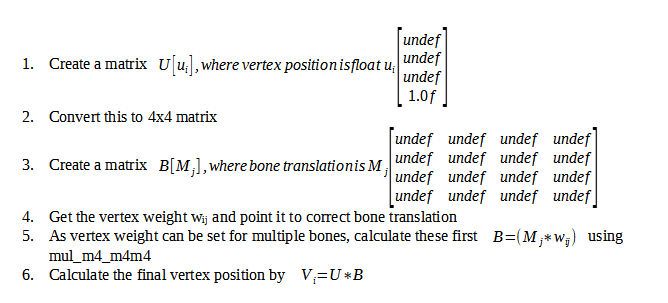# Discussion on improving Corrective smooth modifier

Hello,

I would like to open a discussion on future improvements for Corrective smooth modifier.
On August 2021, a new study was published on Direct Delta Mush Skinning Compression.
This study centers on improving rigging workflow by trying to remove unneccessary manual rigging that is needed on animating a humanoid.

The first study to Delta Mush was introduced in 2014 and was implemented in 2015 as Corrective smooth modifier

As the July 2019 study says on page 113:4 :
“The core idea of Direct Delta Mush schemes. The accumulated affect of iterated Laplacian smoothing is captured in a per-vertex weight mask (column Bi , red color gradient) that if applied to the undeformed geometry produces the equivalent of the iteratively smoothed result (inner blue geometry). These weights are used to solve for the local coordinate transformation Γi which is defined as the best rigid transformation to align the local patch (red regions) from the rest pose to the LBS deformed pose,
a.k.a. a form of Weighted Procrustes problem. The delta between ui and ̃ui is also transformed and added to the smoothed deformed position ̃vi ,producing the final vertex position xi .”

The continued studies to this subjects were introduced in July 2019:

And the newest was released in August 2021:

For reference on different type of skinning methods, kindly check the following:
https://dl.acm.org/doi/10.1145/2897824.2925959

The study also shows that the vertice positions can be calculated using matrix.

I wanted to bring this forward as someone who is interested in new studies for improved workflow on Blender.

For virtual bones, please look at the following presentation:

4 Likes

+1, Corrective Smooth is great, so having ways to improve sounds even greater!1 Like

Improving, yes. But the coding side is a different thing in this situation.

Declare following variables for original corrective smooth modifier:
Let variable u be rest pose that has vertices i
Let the vertex position in rest position be variable ui that is m4, where 4th value is always 1
Concatenate all vectors ui to matrix U, that is matrix 4xn
Deformation of U is driven by LBS model with m bones.
Transformation of the bone j = 1…m is Mj matrix 4x4
Let variable wij be the weight of bone j on vertex i
Assign each vertex (i) to their own bone (j)
Skinned geometry is V = [v1, v2, …, vn] a 4xn matrix, where Vi is computed as wij * Mj * ui
Let variable p be Laplacian smoothing operations
Let the plambda be amount of smoothness
Let the lambda control the precision of the smoothing
Let L be matrix nxn that presents Laplacian matrix of the mesh
Let _L = L * DL ^-1 be the normalized Laplacian, where DL is the diagonal of L
Let _U = [_u1, _u2, …, _un] be the smooth rest pose, a 4xn matrix
Let _V = [_v1, _v2, …, _vn] be the smooth skinned pose, a 4xn matrix
Calculate _U and _V using either implicit method or explicit method.
If lambda is equal or smaller than 1, use explicit method, otherwise use implicit method
Let I be identity matrix nxn with ones on the main diagonal and zeros elsewhere
Explicit method: _U = UA, _V = VA, where A = (I - lambda * _L)^p
Implicit method: _U = UB, _V = VB, where B = (I + lambda * _L)^-p
Recover surface details lost in smoothing using delta variable di, where the difference is computed between original rest pose U and the smooth version _U
[[di],] = ui - _ui
Let final deformed position be xi, that is computed by adding the delta after a local frame transformation Ri, matrix 3x3 to the smooth skinned vertex
xi = _vi + [[Ri * di],]
The local frame transformation Ri is expected to be a rotation matrix, i.e Ri^T * Ri = I and det(Ri) = 1. Alternatively Ri can be approximated from two surface tangent vectors.

The following is the updated smooth modifier / Delta Mush
Direct corrective smooth modifier:
Let Gammai be local rigid transformation, that brings coordinate frame from the rest pose to the deformed position. Therefore Gammai should also bring _ui to _vi
if _vi = Gammai * _ui
xi = Gammai * ui = Gammai * _ui + Gammai(ui - _ui) = Gammai * _ui + [[Ri * di],]

Anyone can correct me as we go through this new method.

What is also interesting here is the use of virtual bones.
This is handled as secondary layer to the normal bones that we use.
User can provide these virtual bones by selecting vertices that act as extra smoothing correction on certain places, where they are needed. This will correct the compression errors/negative bulging, that can easily make extra work during rigging (going through all positions the model might be used for and adding corrections by drive keys).

How these work is that we copy the original weight value to the virtual bone layer and assign that for the vertex. We assign closest vertices to the virtual bone. The weight value is taken from these clusters and moved to the original layer and calculated as average for that bones vertice weights. At the same time scalar weights are updated from the virtual bones to the original layer.If I have understood correctly. Currently in armature_deform.c the position is done as followed:

/* interpolate with previous modifier position using weight group */
if (vert_coords_prev) {
float mw = 1.0f - prevco_weight;
vert_coords[i] = prevco_weight * vert_coords[i] + mw * co;
vert_coords[i] = prevco_weight * vert_coords[i] + mw * co;
vert_coords[i] = prevco_weight * vert_coords[i] + mw * co;
}"

This method could be added as alternative mesh handling for armature modifier. This way you would have the original linear blend skinning and this as secondary. Then you would have behind the corrective smooth this new method as alternative.

This would definitely be cool to have, but isn’t really the same thing as delta mush/corrective smooth from a workflow perspective, it probably should be an option for the armature modifier.

One of the really useful features of corrective smooth is that it doesn’t care at all how the underlying deformation is achieved, so you can stack up all sorts of stuff like shapekeys, shrinkwrap, lattice etc and smooth the combined result.

I agree with you. This is why the armature uses vertex-by-vertex solution and linear blending skinning. But some people might want to have a ready solution with the cost of some resources. The question is, should the whole thing be coded to armature or should it be split to armature and corrective smooth?
How should it behave?